# SAT II Math II : Absolute Value

## Example Questions

### Example Question #11 : Absolute Value

Solve: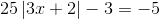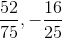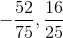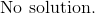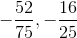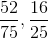Explanation: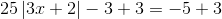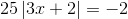Divide by 25 on both sides.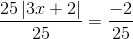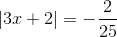Recall that an absolute value cannot have a negative value.  There is no x-value that will equal to the right term.

The answer is:### Example Question #12 : Absolute Value

Solve: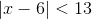.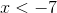or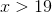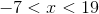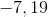Explanation:

Because the absolute value term is "less than" the other side of the equation, we can rewrite the problem like this: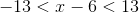This eliminates the absolute value.  Remember, when an operation is performed, it must be performed on all three sets of terms.  When we add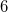to each side, we end up with:### Example Question #13 : Absolute Value

Solve: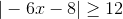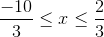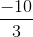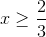or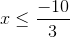orExplanation:

Here, we have to split the problem up into two parts: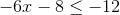and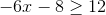First, we can addto each side: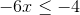Now we divide by -6.  Remember, when you divide by a negative, you flip the sign of the inequality: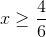Which we can reduce:Now let's do the other part of the problem the same way: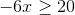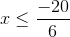### Example Question #14 : Absolute Value

Give the solution set of the inequality: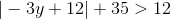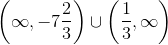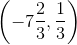All real numbers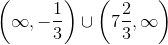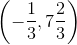All real numbers

Explanation:

To solve an absolute value inequality, first isolate the absolute value expression, which can be done here by subtracting 35 from both sides: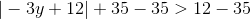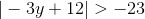There is no need to go further. The absolute value of any number is always greater than or equal to 0, so, regardless of the value of,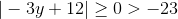.

Therefore, the solution set is the set of all real numbers.

### Example Question #15 : Absolute Value

Solve the absolute value: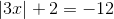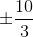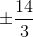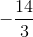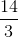Explanation:

Subtract 2 from both sides.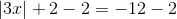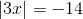Under this condition, there are no values ofthat will give a negative 14.

The answer is:### Example Question #16 : Absolute Value

Solve: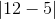Explanation:

The lines on the outside of this problem indicate it is an absolute value problem. When solving with absolute value, remember that it is a measure of displacement from 0, meaning the answer will always be positive.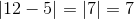For this problem, that gives us a final answer of 7.

### Example Question #80 : Sat Subject Test In Math Ii

Solve: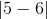Explanation:

The lines on the outside of this problem indicate it is an absolute value problem. When solving with absolute value, remember that it is a measure of displacement from 0, meaning the answer will always be positive.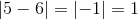For this problem, that gives us a final answer of 1.

### Example Question #17 : Absolute Value

Solve: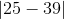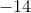Explanation:

The lines on the outside of this problem indicate it is an absolute value problem. When solving with absolute value, remember that it is a measure of displacement from 0, meaning the answer will always be positive.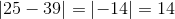For this problem, that gives us a final answer of 14.

### Example Question #82 : Sat Subject Test In Math Ii

Solve: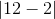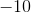Explanation:

The lines on the outside of this problem indicate it is an absolute value problem. When solving with absolute value, remember that it is a measure of displacement from 0, meaning the answer will always be positive.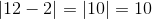For this problem, that gives us a final answer of 10.

### Example Question #11 : Absolute Value

Solve: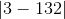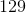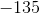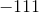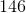Explanation:

The lines on the outside of this problem indicate it is an absolute value problem. When solving with absolute value, remember that it is a measure of displacement from 0, meaning the answer will always be positive.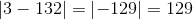For this problem, that gives us a final answer of 129.

### All SAT II Math II Resources